DISCOUNTED CASHFLOW VALUATION

* General Framework for Discounted Cash flow Valuation

* The Dividend Discount Model

- Framework

- Estimating the required rate of return

- Estimating dividends per share

- Estimating terminal price

- Present value vs. Market value

* The Free Cashflow to Equity Model

- Dividends vs. FCFE

- NCR Valuation

- Why the values are different under the two models

- What makes an LBO different?

- Approaches

- The UAL LBO

* Valuing a Restructuring

- Restructuring effects on Growth/Risk

- Valuation Consequences

DISCOUNTED CASHFLOW VALUATION

Basis for Approach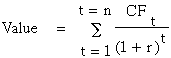where,

n = Life of the asset

CFt = Cashflow in period t

r = Discount rate reflecting the riskiness of the estimated cashflows

Equity Valuation versus Firm Valuation

Two basic sub-approaches --

* value just the equity stake in the business

* value the entire firm, which includes, besides equity, the other claimholders in the firm

I.Equity Valuation

The value of equity is obtained by discounting expected cashflows to equity, i.e., the residual cashflows after meeting all expenses, tax obligations and interest and principal payments, at the cost of equity, i.e., the rate of return required by equity investors in the firm.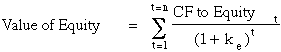where,

CF to Equityt = Expected Cashflow to Equity in period t

ke = Cost of Equity

The dividend discount model is a specialized case of equity valuation, and the value of a stock is the present value of expected future dividends.

II. Firm Valuation

The value of the firm is obtained by discounting expected cashflows to the firm, i.e., the residual cashflows after meeting all operating expenses and taxes, but prior to debt payments, at the weighted average cost of capital, which is the cost of the different components of financing used by the firm, weighted by their market value proportions.where,

CF to Firmt = Expected Cashflow to Firm in period t

WACC = Weighted Average Cost of Capital

FIRST LAW OF VALUATION

Never mix and match cash flows and discount rates. The key error to avoid is mismatching cashflows and discount rates, since discounting cashflows to equity at the weighted average cost of capital will lead to an upwardly biased estimate of the value of equity, while discounting cashflows to the firm at the cost of equity will yield a downward biased estimate of the value of the firm.

THE DIVIDEND DISCOUNT MODEL

The value of a stock in the dividend discount model is the present value of expected dividends over time, discounted at a rate that is appropriate for the riskiness of the expected cashflows. There are many versions of the dividend discount model in use. We will consider only the most widely used - the two stage dividend discount model.

Two-Stage Dividend Discount Model

The Model:

The model is based upon two stages of growth, an extraordinary growth phase that lasts n years, and a stable growth phase that lasts forever after that:

Extraordinary growth rate: g% each year for n years ............Stable growth: gn forever

|____________________________________________|____________________>

Value of the Stock = PV of Dividends during extraordinary phase + PV of terminal price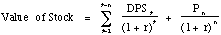where

DPSt = Expected dividends per share in year t

r = Required rate of return

Pn = Price at the end of year n

There are four basic inputs needed for the dividend discount model-

* Time Horizon for Extraordinary Growth (n): This is the period of extraordinary growth. It will depend upon the firm being valued. The higher the current growth rate and the more volatile the business a firm is in, the longer the extraordinary growth period should be. As a rule of thumb,

If current growth rate is Extraordinary growth period

< 7%....... 0 years

7 - 15%... 5 years

> 15%..... 10 years

* Discount Rate (r): The cash flows that flow to equity investors should be discounted at the cost of equity. (Remember the first principle of discounting.) The cost of equity, in turn, will be a function of the riskiness of the stock. If the CAPM is used,

ke = Rf + b (E(RM) - Rf)

where,

ke = Cost of Equity

Rf = Riskfree Rate

b = Beta of the stock

E(RM) - Rf = Risk Premium for investing in risky investments

* Expected Dividends (DPSt) : The expected dividends can be obtained by taking current dividends and applying an expected growth to it. The better approach is to start with current earnings.

Step 1: Estimate current earnings per share

Step 2: Estimated expected growth in earnings (g) during the extraordinary growth period.

Step 3: Estimate expected earnings per share in future periods

Step 4: Estimate dividend payout ratios for each of the future periods.

Step 5: DPSt = EPSt (1+g)t (Dividend Payoutt)

* Terminal Price (Pn): The terminal price is the expected stock price at the end of the high growth period. It can be estimated in one of two ways -

* Infinite Growth Model

Assumption: The growth rate after the high growth period will be stable and remain constant forever after that. (gn = Stable growth rate after year n)

Model: The terminal price, if the growth is stable after year n, can be written as -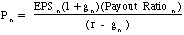What is a stable growth rate?

As a general rule of thumb, this infinite growth rate cannot exceed the overall growth rate for the overall economy (GNP) by more than a small amount (2-3%). The growth rate in the overall economy can be obtained fairly simply by adding the core long term inflation rate to the long term growth rate in real GNP.

Estimate for the US

Upper end: Long term inflation rate (5%) + Growth rate in real GNP (3%) =8%

Lower end: Long term inflation rate (3%) + Growth rate in real GNP (2%) = 5%

With this estimate, the infinite growth rate cannot be greater than 10% (8%+2%) for the upper end of the spectrum or 7% (5%+2%) for the lower end of the spectrum.

1. You are valuing a company which is expected to have a great deal of growth from international expansion. How would that affect your estimates of growth during the stable growth period?

( ) I would not make any adjustment

( ) I would increase the stable growth rate substantially

( ) I would use a longer growth period and use the same stable growth rate

( ) I would not adjust the high growth period, and use a marginally higher stable growth rate.

( ) I would use a longer growth period and a marginally higher stable growth rate.

What is the ceiling on the expected growth?

What is a reasonable payout ratio for the terminal price calculation?

The payout ratio after the high growth period is likely to be different from the payout ratio during the period. Intuitively, if the growth rate is expected to decline significantly after year n. There are a couple of ways of estimating this payout ratio -

(a) Use an average payout ratio for a stable firm (generally between 50% and 70%)

(b) Use the fundamental growth rate formula to estimate a reasonable payout ratio, given the stable growth rate. (See section on fundamental growth)

* Price-Earnings Ratio

Assumption: The stock will trade at the same price-earnings ratio as the rest of the industry by the end of the high growth period.

Model:

Pn = EPSn * Average Price/Earnings Ratio for the Industry

A Simplified Version of the Dividend Discount Model

In the case where the extraordinary growth rate (g) and payout ratio are the same for the first n years, this formula can be simplifed as follows: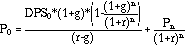where Pn is the terminal price as defined above. This simplifies calculations because it does not require the estimation of dividends each year for the first n years.

More On Estimating Earnings Growth
I. Historical Growth Rates

A. Arithmetic Average versus Geometric Average

The average growth rate can be very different depending upon whether it is an arithmetic average or a geometric average. The arithmetic average is the mean of past growth rates, while the geometric mean takes into account the compounding effect. The latter is clearly a much more accurate measure of true growth in past earnings, especially when year-to-year growth has been erratic. This can be illustrated with a simple example.

B. The Effect of Size on Growth

As firms get larger, it becomes more and more difficult to sustain high growth rates in earnings.

II. Earnings Growth and Fundamentals

A. Earnings Growth and Return on Equity

Derivation: Define E0 to be current earnings and _ E to be the change in earnings next year. Also define I to be the amount invested back in the company. Then,

Earnings Growth rate = _ E / E0

= (I/ E0) * (_ E / I)

= (1 - payout ratio) * (ROE)

= b* ROE

Implications: The earnings growth rate of a company is an increasing function of

- the amount reinvested back in the company, i.e. retention ratio 'b'

- the quality of investments in which the money is reinvested, i.e. ROE.

2. You attempting to estimate expected growth for The Gap and J.P. Morgan. The Gap has a return on equity of 25% and pays out 30% of its earnings as dividends. J.P. Morgan has a return on equity of 15% and pays out 50% of its earnings as dividends. Estimate the expected growth rate for each of these companies ñ

( ) The Gap's expected growth =

( ) J.P. Morgan's expected growth =

What is the ceiling on the expected growth?

B. Return on Equity and Leverage

The return on equity, and by implication, the growth rate, are affected by the leverage decisions of the firm. In the broadest terms, increasing leverage will lead to a higher return on equity if the pre-interest, after-tax return on projects (assets) exceeds the after-tax interest rate paid on debt. This is captured in the following formulation of return on equity:

ROE = ROA + D/E (ROA - i (1-t))

where,

ROA = (Net Income + Interest (1 - tax rate)) / (BV of Debt+BV of Equity)

= EBIT (1- t) / BV of Debt and Equity

D/E = Debt/ Equity (Market Value if available)

i = Interest Expense on Debt / BV of Debt

t = Tax rate on ordinary income

The derivation is simple and is provided in a footnote. Using this expanded version of ROE, the growth rate can be written as:

g = b (ROA + D/E (ROA - i (1-t))

The advantage of this formulation is that is allows explicitly for changes in leverage and the consequent effects on growth. It is a useful way of analyzing the effects of restructuring on growth and value.

3. Assume that you estimating the effect of a recent restructuring at Rubbermaid on expected growth. Rubbermaid has a return on assets of 18%, has no leverage and pays out 20% of its earnings as dividends. It is planning to sell of low-return assets and increase its return on assets to 20%, increase its debt equity ratio to 25% and pay 30% of its earnings as dividends. The tax rate is 40%, and the pre-tax borrowing rate is 10%. Estimate the expected growth before and after the restructuring.

( ) E(growth) before restructuring =

( ) E(growth) after restructuring =

Does the higher growth automatically mean that the value of the stock will increase?

( ) Yes

( ) No

Estimating Payout Ratio for Stable Growth Period

The payout ratio after the high growth period can be estimated, based upon the stable growth rate and the fundamentals of the firm.

Payout Ratio during Stable Phase = 1 - (gn/ (ROA + D/E (ROA-i(1-t)))

where,

gn = Stable Growth Rate

ROA = Return on Assets during stable growth period

D/E = Debt / Equity Ratio

i = Interest rate on debt

4. Consider the example of Rubbermaid described above. Given the ROA of 20%, the debt equity ratio of 25% and the pre-tax cost of debt of 10%, estimate the payout ratio that Rubbermaid can afford to have, given the expected growth rate of 6%.

r Payout Ratio in Stable Phase =

Why is the payout ratio so high?

III. Analyst Forecasts of Growth

How good are analysts at forecasting earnings?

Box- Jenkins: is a simple time series model for forecasting earnings per share using past EPS.

Studies seem indicate that analysts are better at forecasting earnings than simple time series models.

The Accuracy of Short Term Forecasts

The general consensus from studies that have looked at short-term forecasts (one quarter ahead to four quarters ahead) of earnings is that analysts provide better forecasts of earnings than models that depend purely upon historical data. The following table summarizes the results from three studies of analysts forecasts.

Mean Relative Absolute Error

Study Analyst Group Analyst Mechanical

Forecasts Models

Collins & Hopwood Value Line Forecasts 31.7% 34.1%

1970-74

Brown & Rozeff Value Line Forecasts 28.4% 32.2%

1972-75

Fried & Givoly Earnings Forecaster 16.4% 19.8%

1969-79

The mean relative absolute error measures the absolute difference between the actual earnings and the forecast for the next quarter, in percentage terms. The mechanical models are all based upon historical data.

* Does The Dividend Discount Model Work?

Study inputs:

Current EPS = Average of last five years of earnings

Discount rate = CAPM adjusted return

Intermediate growth rate = IBES Analysts forecasts of earnings growth

Terminal growth rate = 8 % for all stocks

Payout ratio = Set equal to 45 % even for companies that do not pay dividends

Results: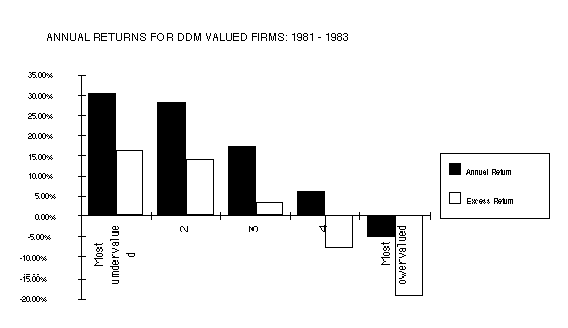THE FREE CASHFLOW TO EQUITY MODEL

A. FCFE vs Dividends

FCFE is what the company has available to pay out as dividends. The free cashlfow to equity is the cashflow left over after working capital and capital investment needs:

Item Assumptions

Net Income : Net profit after taxes

- (Cap Ex - Deprec'n) * (1 - DR) : Capital investment needs * (1 - Debt Ratio)

- Chg in Working Capital * (1 - DR) : D Working Capital * (1 - Debt Ratio)

= Free Cashflows to Equity : Cashflows available to equity investors

B. The Two-stage Model

The value of any stock is the present value of the FCFE per year for the extraordinary growth period plus the present value of the terminal price at the end of the period.

Value = PV of FCFE + PV of terminal price

= Sum of FCFEt / (1+r)t + Pn / (1+r)n

where,

FCFEt = Free Cashflow to Equity in year t

Pn = Price at the end of the extraordinary growth period

r = Required rate of return to equity investors in the firm

The terminal price is generally calculated using the infinite growth rate model,

Pn = FCFEn+1 / (r - gn)

where,

gn = Growth rate after the terminal year forever.

C. Calculating the terminal price

If infinite growth rate model used:The same caveats that apply to the growth rate for the Gordon Growth Rate model (within 2% of growth rate in nominal GNP) apply here as well. In addition, the free cashflow to equity after the terminal year has to be consistent with this assumption of stable growth rate. A strong implication of this is that capital spending and depreciation have to be in rough parity. (Some even suggest that they should offset each other) A stable firm cannot be spending twice as much on equipment each year as it is getting back from depreciation.

5. Many analysts assume that capital expenditures offset depreciation, when doing valuation. Is it an appropriate assumption to make for a high growth firm?

( ) Yes

( ) No

What about a stable growth firm?

( ) Yes

( ) No

VALUING NCR USING DIVIDEND DISCOUNT MODEL AND THE FCFE MODEL

Step 1: Estimate the required rate of return for stockholders
 Beta= 1.15 T.Bond Rate = 9.00% Exp. Return = 15.33% Market risk premium = 5.50%

Step 2: Estimate the current EPS, the expected growth rate in earnings and expected dividends per share.

Current Earnings per Share = \$5.43

Dividend Payout Ratio = 26.00% Retention Ratio = 74%

Return on Assets

= (Net Income + Interest Expenses (1-tax rate) / (BV: Debt + BV: Equity)

= ( \$369 + \$40 (1-0.34))/(537+1850) = 16.56%

Debt Equity Ratio = Debt/Market Value of Equity = 537/(88*67.96)

= 8.98%

Interest Rate on Debt = 7.45%

Expected Growth Rate in Earnings

= Retention Ratio ( ROA + D/E (ROA - i (1-t))

= 0.74 (16.56% + .0898 (16.56% - 7.45% (1-0.34))

= 13.03%

[In comparison, the historical growth rate was 11.51% and the analyst projection was 9.50%]
 Year EPS DPS 1 \$6.14 \$1.60 2 \$6.94 \$1.80 3 \$7.84 \$2.04 4 \$8.86 \$2.30 5 \$10.02 \$2.60

Step 3: Estimate the current capital expenditures, depreciation and working capital needs and expected future growth in each.

Current Revenues per share = \$92.60

Current Capital Expenditures = \$6.20 Current Depreciation = \$4.41

Working Capital as a percent of revenues = 15% (Averaging 1990-92 numbers)

Debt Financing used to finance investment needs = 537/(537 + 88*67.96) = 8.24%
Capital expenditures, depreciaton and revenues are expected to grow at the same rate as earnings (13.03%).
 Year Depreciation Capital Expenditures (Cex-Depr)(1-_) D WC (1-_) 1 \$4.99 \$7.01 \$1.85 \$1.66 2 \$5.64 \$7.92 \$2.09 \$1.88 3 \$6.38 \$8.95 \$2.37 \$2.12 4 \$7.21 \$10.12 \$2.67 \$2.40 5 \$8.15 \$11.44 \$3.02 \$2.71

_ = Debt Ratio = 8.24%

The FCFE = EPS - (Capital Expenditures - Depreciation) (1-_) - D Working Capital (1-_)
 Year EPS (Cex-Depr)(1-_) D WC (1-_) FCFE/sh 1 \$6.14 \$1.85 \$1.66 \$2.63 2 \$6.94 \$2.09 \$1.88 \$2.97 3 \$7.84 \$2.37 \$2.12 \$3.36 4 \$8.86 \$2.67 \$2.40 \$3.79 5 \$10.02 \$3.02 \$2.71 \$4.29

6. Based upon the FCFE, what proportion of the earnings can be paid out as a dividend?

( ) Dividend Payout Ratio =

Step 4: Estimate the terminal price

The infinite growth model is used to value NCR.

Growth rate after year 5 = 6%

Beta after year 5 = 1.00

ROA after year 5 = 15.00%

D/E ratio and interest rate are expected to remain unchanged.

Expected Payout Ratio after year 5

= 1 - Expected Growth Rate/ ( ROA + D/E (ROA - i (1-t))

= 1 - 6% / (.15 + 0.0898 (.15 - .0745 (1-.34)) = 62.28%

Terminal Price from the Dividend Discount Model

= EPS5 * (1+gn) * Stable Payout Ratio / (r - gn)

= \$10.02(1.06)(.6228)/(.145-.06) = \$ 77.81

Capital Expenditures are expected to offset depreciation after year 5

Working Capital will continue to remain at 15% of Revenues

FCFE in year 6 = EPS6 - (Capital Expenditures6 - Depreciation6)(1-_) - D WC(1-_)

= \$10.02(1.06) - \$0 - \$1.54(1-.0824) = \$9.21

D WC =[ (Revenues6 - Revenues5)*WC as % of Revenues]/Number of Shares = \$1.54

Terminal Price = \$9.21/(.145-.06) = \$ 108.36

Step 5 : Calculate the present value
 Year DPS TERM. PR. FCFE/sh Term. Val. 1 \$1.60 \$2.63 2 \$1.80 \$2.97 3 \$2.04 \$3.36 4 \$2.30 \$3.79 5 \$2.60 \$77.81 \$4.29 \$108.36

Present Value (DDM)

= 1.60/1.1533 + 1.80/(1.1533)2 + 2.04/(1.1533)3 + 2.30/(1.1533)4+ (2.60+77.81)/(1.1533)5 = \$44.79

Present Value (FCFE)

= 2.63/1.1533 + 2.97/(1.1533)2 + 3.36/(1.1533)3 + 3.79/(1.1533)4+ (4.29+108.36)/(1.1533)5 = \$64.06

7. The dividend discount model provides an estimate of value that is almost \$20 lower per share than the FCFE model. Which of the following statements would you most agree with?

( ) The DDM Value will always be lower than the FCFE value

( ) The FCFE Value will generally by higher than the DDM

( ) Firms which pay out much less in dividends than they have available in FCFE will generally have higher FCFE values than DDM values.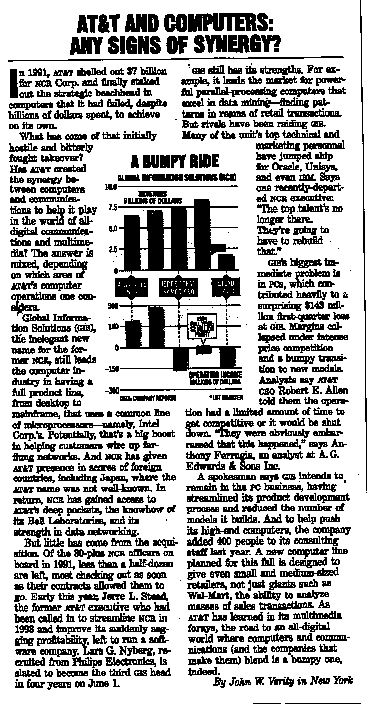FCFE Valuation versus Dividend Discount Model Valuation

a. When they are similar

* Where the dividends are equal to the FCFE

* Where the FCFE is greater than dividends, but the excess cash (FCFE - Dividends) is invested in projects with net present value of zero.

b. When they are different

When dividends are less than FCFE

* When the FCFE is greater than the dividend and the excess cash either earns below-market interest rates or is invested in negative net present value projects, the value from the FCFE model will be greater than the value from the dividend discount model.

* The payment of smaller dividends than can be afforded to be paid out lowers debt-equity ratios and may lead the firm to become underleveraged, causing a loss in value.

When dividends are greater than FCFE

* Flotation cost on new equity issues creates an expense which decreases value.

* If the firm borrows the money to pay the dividends, the firm may become overleveraged (relative to the optimal) leading to a loss in value.

* Paying too much in dividends can lead to capital rationing constraints where good projects are rejected, resulting in a loss of wealth.

c. What does it mean when they are different?

* Difference between the value from the FCFE model and the value using the dividend discount model can be considered one component of the value of controlling a firm - it measures the value of controlling dividend policy.

• In more infrequent case, where the value from the dividend discount model exceeds the value from the FCFE, the difference has less economic meaning but can be considered a warning on the sustainability of expected dividends.

VIII & IX. TWO AND THREE STAGE VERSIONS OF THE FCFF MODEL

The Model

The value of the firm, in the most general case, can be written as the present value of expected free cashflows to the firm:

Value of Firm =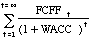where,

FCFFt = Free Cashflow to firm in year t

WACC = Weighted average cost of capital

If the firm reaches steady state after n years, and starts growing at a stable growth rate gn after that, the value of the firm can be written as:

Value of Firm =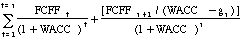Firm Valuation versus Equity Valuation

• The value of equity, however, can be extracted from the value of the firm by subtracting out the market value of outstanding debt.
• The advantage of using the firm valuation approach is that cashflows relating to debt do not have to be considered. In cases where the leverage is expected to change significantly over time, this is a significant saving. The firm valuation approach does, however, require information about debt ratios and interest rates to estimate the weighted average cost of capital.
• The value for equity obtained from the firm valuation and equity valuation approaches will be the same if:

(b) Bonds are correctly priced

Best suited for:

• Firms which have very high leverage and are in the process of lowering their leverage or vice versa.
• Firms which have negative FCFE, but have positive FCFF.

Illustration: Federated Department Stores: Valuing an over-leveraged firm using the FCFF approach

A Rationale for using the Two-Stage FCFF Model

• The earnings before interest and taxes at Federated in 1994, which amounted to \$531 million, were still well below EBIT in 1988 of \$628 million. The earnings are expected to grow at rates slightly above-stable for the next five years as the firm recovers.
• The leverage in 1994 was still significantly above desirable levels, largely as a consequence of the leveraged buyout in the late eighties. It was anticipated that this debt ratio would be lowered gradually over the next five years to acceptable levels.

Background Information

• Base Year Information
• Earnings before interest and taxes in 1994 = \$ 532 million
• Capital Expenditures in 1994 = \$310 million
• Depreciation in 1994 = \$207 million
• Revenues in 1994 = \$ 7230 million
• Working Capital as percent of revenues = 25.00%
• Tax rate = 36%
• High Growth Phase
• Length of High Growth Phase = 5 years
• Expected Growth Rate in FCFF = 8%
• Financing Details
• Beta during high growth phase = 1.25
• Cost of Debt during high growth phase = 9.50% (pre-tax)
• Debt Ratio during high growth phase = 50%
• Stable Growth Phase
• Expected growth rate in FCFF = 5%
• Financing Details
• Beta during stable growth phase = 1.00
• Cost of Debt during stable growth phase =8.50%
• Debt Ratio during stable growth phase = 25%
• Capital expenditures are offset by depreciation.

Valuation

The forecasted free cashflows to the firm over the next five years are provided below:
 1 2 3 4 5 Terminal year EBIT \$574.45 \$620.41 \$670.04 \$723.64 \$781.54 \$820.61 - t (EBIT) \$ 206.80 \$223.35 \$241.21 \$260.51 \$281.35 \$295.42 - (Cap Ex - Depreciation) \$111.24 \$120.14 \$129.75 \$140.13 \$151.34 \$0.00 - Ch Working Capital \$144.58 \$156.15 \$168.64 \$182.13 \$196.70 \$132.77 = FCFF \$101.83 \$120.77 \$130.44 \$140.87 \$152.15 \$392.42

Cost of Equity during high growth phase = 7.5% + 1.25 (5.5%) = 14.38%

Cost of Capital during high-growth phase = 14.38 % (0.5) + 9.50 % (1-0.36) (0.5) = 10.23%

The free cashflow to the firm in the terminal year is estimated to be \$392.42 million.

FCFF in terminal year = EBIT6 (1-t) - (Rev6-Rev5)*Working Capital as % of Revenue

= \$ 820.61 (1-0.36) - \$ 132.77 = \$ 392.42 millions

Cost of Equity during stable growth phase = 7.50% + 1.00 (5.50%) = 13.00%

Cost of Capital in stable growth phase = 13.00% (0.75) + 8.50% (1-0.36) (0.25) = 11.11%

Terminal value of the firm = \$ 392.42 / (.1111 - .05) = \$ 6,422 millions

The value of the firm is then the present value of the expected free cashflows to the firm and the present value of the terminal value:
 PV of FCFF \$487.17 PV of Terminal Value = \$3,946.93 Value of Firm = \$4,434.11 Value of Debt = \$2,740.58 Value of Equity = \$1,693.52 Value Per Share = \$13.38

Federated Department Stores was trading at \$21 per share in March 1995.

SPECIAL CASES IN VALUATION

* Cyclical or Troubled Firms: There are two general problems in valuing these firms-

- Current earnings are generally depressed and in many cases negative. When earnings are depressed, using them in valuation will result in very low values. When they are negative, it becomes even more difficult.

- Historical growth rates are negative and often meaningless.

In these cases, earnings have to be normalized. There are a number of different ways of doing this -

(a) If earnings are depressed purely because of the economic cycle, and are expected to rebound once the economy recovers:

* The standard approach is to average income over a period long enough to include an economic recovery and a recession.

* A variant on this approach that allows for scaling to size is to calculate the average return on equity or return on assets over the period. This return on equity is applied to the current book value of equity to arrive at a standardized net income. Alternatively, the return on assets is applied to the current book value of assets to arrive at a standardized after tax operating income.

Example: Ford Motor Company reported earnings per share of -\$0.73 in 1992, in the midst of the recession. The following are estimated normalized earnings per share using both the average income and average r